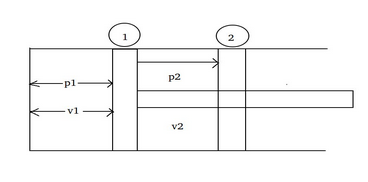# Amount of Work done by the system.

## Consider a piston cylinder arrangement with gas filled in cylinder. If the piston moves from position 1 to position 2 as shown in figure with volume changes from v1 to v2, the amount of work W by the system will be,a. v1v2 p dV
b. v1v2 p V
c. v1v2 v dp
d. none of the above

Correct Answer : a. v1v2 p dV

Explanation :

Initially at position 1, let the pressure and volume be p1 and V1 respectively. The piston is the only boundary which moves with gas pressure. Let the piston move out to a new final position 2. The pressure and volume will be p2 and V2 respectively. At any intermediate point let the pressure be p and volume be V. These macroscopic properties are significant for equilibrium state. When the piston moves as infinitesimal distance dl, and area of the piston is 'a', the force F acting on the piston F = p*a and the infinitesimal amount of work done by the gas will be,
dW = F * dl = padl = pdV